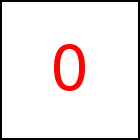# vBegin Library Method

Short Description: Set initial velocity

Signature: t.vBegin (x,y,z) or (v)
Group: Motion
Class: transition Class

Implicitely sets the acceleration used for a motion with constant acceleration by specifying the inital velocity of the object at the beginning of the transition. The acceleration is calculated so that the object has the specified initial velocity and that it reaches the specified end point of the motion in specified the duration. Please refer to Motions for an explanation of motion with constant acceleration. Default acceleration is zero.

Sample HTML element with id="sample"

If the velocity is given in scalar form it works in the direction of the motion (or against it in case v<0) with magnitude v. In this case either starting or end point or both have to be set (e.g. using from or to) and must not be identical.

Duration needs to be set before using this method. Also note that the acceleration may be set just once using one of the functions acceleration, vBegin and vEnd.

 taccgl.actor("sample") . to({oy:500}). vBegin(0) . dur(5). start() RUN taccgl.actor("sample") . to({oy:500}). vEnd(0) . dur(5). start() RUN taccgl.actor("sample") . to({oy:500}). vBegin(1000) . dur(1). start() RUN taccgl.actor("sample") . to({oy:500}). vBegin(1000) . dur(5). start() RUN taccgl.actor("sample") . to({oy:500}). vBegin(0,1000,0) . dur(5). start() RUN taccgl.actor("sample") . to({oy:500}). vBegin(-1000) . dur(5). start() RUN

Acceleration and velocity can as well be given in vector form. The examples below show various values and directions for the initial velocity and the acceleration.

 taccgl.actor("ball") . dur(2). vBegin(0,-500,0). start() RUN taccgl.actor("ball") . to({ox:-500}). dur(2). vBegin(0,-500,0). start() RUN taccgl.actor("ball") . to({ox:-500}). dur(2). vBegin(500,-500,0). start() RUN taccgl.actor("ball") . to({ox:-500}). dur(2). vBegin(-500,-500,0). start() RUN

WebGL™ is a trademark of the Khronos Group Inc.

Next Page:transition.vEnd - Set final velority
Previous Page: transition.acceleration - Specify Acceleration as Vector

# Motion

taccgl™ - Canvas 3D Animation Library